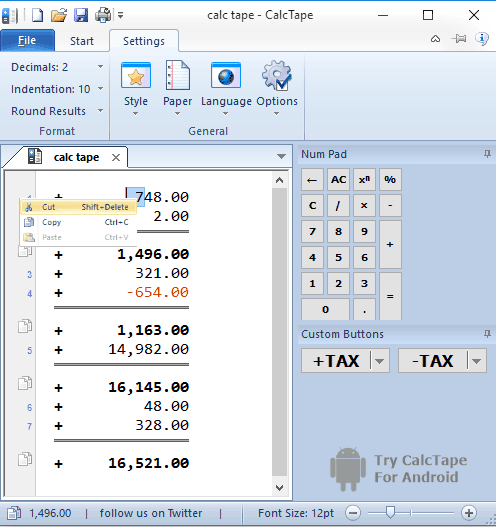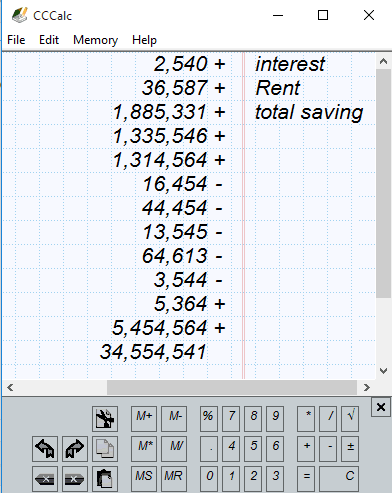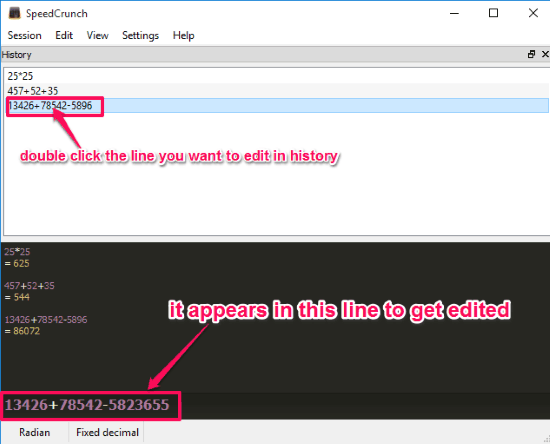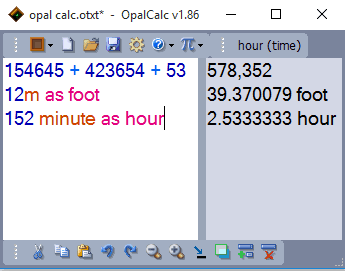Editor Ratings:
User Ratings:
[Total: 0 Average: 0]

Here are 5 best free Check and Correct calculators for Windows. Unlike default calculator of Windows, these calculator not only show the history of our previous calculations but they also allow us to edit them. With these calculators you can also save your calculations to the PC. These saved files can later be loaded and edited in their respective calculators. Some of these calculators are for simple calculations only, while some even offer complicated and scientific calculations. Some of them even offer basic conversions like Meter to Foot, Dollars to Pound, Minutes in Hours, etc.

Let’s see what these free check and correct calculators offer.

## CalcTapeCalcTape is a free check and correct calculator for Windows. It is basically very useful, as it allows you to edit previous calculations, so you won’t have to repeat calculations if there is some error. It is a basic calculator software which allows us to do simple calculations. However it also provides 2 custom buttons which you can set to add and subtract a constant % like sales tax, service tax, etc. You can also round up the sum if they are in decimal. You can also round up larger decimal number from 0 to 8 decimal places. You can also save your calculations as “.calc” files on your computer. It also allows you to print your active calculations directly from your calculator. You can also use cut, copy, and paste with this calculator.

## CCCalcCCCalc is a very simple and intuitive Calculator for Windows. This also works as a free check and correct calculator for Windows. With this calculator you can edit errors in your calculations, which saves a lot of time. This calculator also allows you to save calculations on your PC, however I was not able to to load the saved calculation file. You can try it for yourself; who knows it might work for you.

It allows you to use Cut, Copy, Paste, Undo, and Redo. You can add comment to every calculation step if you want. This calculator is also meant for basic mathematical calculations. You can either use your keyboard for calculation or you can use keyboard integrated with the calculator. It also allows to roundup decimals according to your need.

##(adsbygoogle = window.adsbygoogle || []).push({});

Speed Crunch is a useful check and correct calculator. It is also a basic mathematical calculator used for basic arithmetic calculations. It also allows you to edit your mathematical calculations. You can only edit calculations in this calculator by opening History and selecting the calculation you want to edit. History can be opened from View tab on the interface.

It also has list of universal constants and also a list of basic formulas of mathematics. Speed Crunch also allows you to save calculations to your desktop. You can load the saved calculation back on the calculator anytime. It also allows to export calculations as HTML to your PC.

##Precise Calculator is a scientific cum engineering calculator which also acts as a check and correct calculator. It allows you to edit any ongoing calculation very easily, as you just need to click where you want to edit it.

You can easily save your calculations to the desktop. However you won’t be able to load the calculation back on the calculator as the calculator saves the calculation as a TXT file.

Since it a scientific calculator so you can solve all types of mathematical problems with this calculator. It also has a list of all the units of Length, Area, Volume, Mass, Energy, Power, Pressure, Speed, and Time. So, you can directly convert units within this calculator. It also has a list of most used universal constants.

## Opal CalcOpal Calc is a new type of calculator which allows you to use natural language into the calculation, making it more interesting. It also allows you to edit previous calculation. For that you just need to click where you want to edit, and the calculation automatically adjusts according to the new entry.

It also converts Distance, Currency, Time, Weight, etc. You can also use built in Operators, Functions, Special vars, and Constants, during calculations. It automatically gives different colors to different operators so the equation can always be easily readable. It also allows to use Cut, Copy, Paste, Undo, and Redo in the calculation

The free version of this amazing calculator only allows us to use 5 lines of calculations. For calculating more than 5 lines, you will need to pay.

## Verdict

These free check and correct calculators for Windows are very useful, especially if you have to do long calculations. You can easily check each step of your calculation, print the calculation if required, and also fix any mistakes.

Which of these calculators you liked the most? Let me know in comments below.

 Editor Ratings: User Ratings:[Total: 0 Average: 0] Tags: calculator